## Pages

Showing posts with label class6-maths. Show all posts
Showing posts with label class6-maths. Show all posts

# Class 6 Maths - Knowing Our Numbers (Roman Numbers)

👉 Around the 3rd century BCE, Romans developed their own number system for counting.

👉 Roman Numerals used symbols to represent numbers such as

Roman Numeral Hindu-Arabic Numeral

I 1

V

X 10

L 50

C 100

D 500

M 1000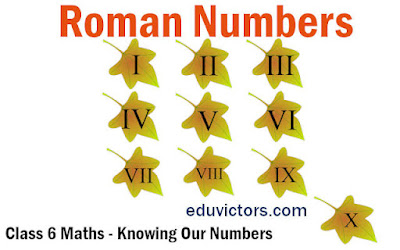# Class 6 Maths - Integers ( Questions and Answers)

Important Points:

The collection of numbers ....−2, −1, 0, 1, 2 .... is called integers.

The numbers with the negative sign are called negative integers and the numbers with positive sign are called positive integers.

One more than the given number gives successor and one less than the given number gives predecessor.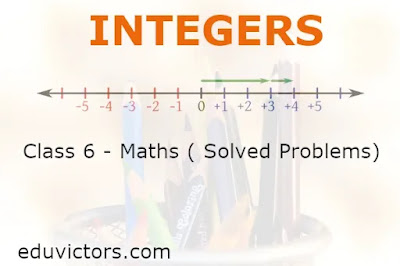# Class 6 - Maths - Playing With Numbers (Assignment)

Fill in the blanks with suitable word(s).

1. Numbers having more than two factors are called _____________ numbers.

2. A _______ of a number is an exact divisor of that number.

3. ______ is a factor of every number.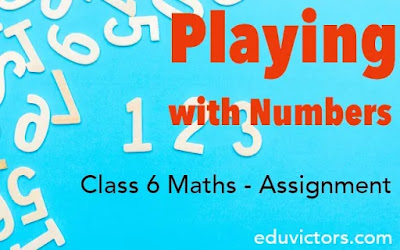# Class 6 - Maths: Integers (Worksheet)

Fill in the blanks in the following sentences with suitable word(s):

1. A collection of – 1, – 2, – 3, – 4, ... is called ________ integers.

2. Every positive integer is ________ than every negative integer.

3. Zero is ______ than every positive integer.## Basic Geometry Ideas - Quiz

Class 6 - Mathematics

Q1: Three or more lines which pass through same point are called

(a) intersecting lines
(b) parallel lines
(c) perpendicular lines
(d) concurrent lines.

Q2: Geometrical figure which has no dimension is

(a) line
(b) plane
(c) line segment
(d) point.

Q3: The lines which do not intersect and have equal distance between them are called:

(a) parallel lines
(b) perpendicular lines
(c) intersecting lines
(d) straight lines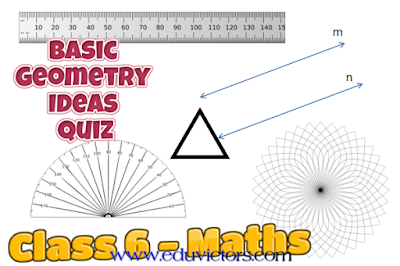## Number System

Multiple Choice Questions (MCQs)

Question 1: The product of the place values of two 2’s in 428721 is

(a) 4
(b) 40000
(c) 400000
(d) 40000000

Question 2:  3 × 10000 + 7 x 1000 + 9 x 100 + 0 x 10 + 4 is the same as

(a) 3794
(b) 37940
(c) 37904
(d) 379409

Question 3: If 1 is added to the greatest 7-digit number, it will be equal to

(a) 10 thousand
(b) 1 lakh
(c) 10 lakh
(d) 1 crore## Chapter- 10:  Mensuration (Practice Sheet)

Q1. Find the perimeter of the following:

(a) A rectangle of length 16cm and breadth 10cm.
(b) A parallelogram of length 21 cm and breadth 15cm.
(c) A triangle with sides measuring 10cm,14cm and 16cm.

Q2. How much does Shamim have to walk to go around a square field of side 10m?

Q3. What is the length of a coloured tape required to stick on the sides of a rectangular scenery. The length of the scenery is 20 cm and breadth is 12cm.

Q4. How much wire is required to fence a rectangular field 1 km long and 500m broad?

Q5. The area of a playground is 1547 sqm. Its breadth is 17m. What is the length of the playground?## Playing With Numbers  (Natural Numbers and Whole Numbers)

Worksheet

Fill in the blanks

1. The set of numbers 1, 2, 3, 4... are called n________ numbers or c_________ numbers.

2. The smallest whole number is ________

3. The smallest natural number is ________

4. There is ______ greatest number(s) in the number system. (many/no/one)## Friday 5 May 2017

### CBSE Class 6 Maths - Playing With Numbers - NCERT Exercise 3.7 (#cbsenotes)

Playing With Numbers

## EXERCISE 3.7

CBSE Class 6 Maths

Q1: Renu purchases two bags of fertiliser of weights 75 kg and 69 kg. Find the maximum value of weight which can measure the weight of the fertiliser exact number of times.

```Answer:
The maximum weight  = HCF of 75 and 69.
Factors of 75   = 3 ✕ 5 ✕ 5
Factors of 69   = 3 ✕ 69
HCF  = 3
∴ the required weight is 3 kg.
```

Q2: Three boys step off together from the same spot. Their steps measure 63 cm, 70 cm and 77 cm respectively. What is the minimum distance each should cover so that all can cover the distance in complete steps?## Tuesday 2 May 2017

### CBSE Class 6 Maths - Playing With Numbers - NCERT Exercise 3.6 (#cbsenotes)

Playing With Numbers

## EXERCISE 3.6

CBSE Class 6 Maths

Q1: Find the HCF of the following numbers:
(a) 18, 48
(b) 30, 42
(c) 18, 60
(d) 27, 63
(e) 36, 84
(f) 34, 102
(g) 70, 105, 175
(h) 91, 112, 49
(i) 18, 54, 81
(j) 12, 45, 75

(a) Factors of 18 = 2 × 3 × 3
Factors of 48 = 2 × 2 × 2 × 2 × 3
HCF (18, 48) = 2 × 3 = 6

(b) Factors of 30 = 2 × 3 × 5
Factors of 42 = 2 × 3 × 7
HCF (30, 42) = 2 × 3 = 6

(c) Factors of 18 = 2 × 3 × 3
Factors of 60 = 2 × 2 × 3 × 5
HCF (18, 60) = 2 × 3 = 6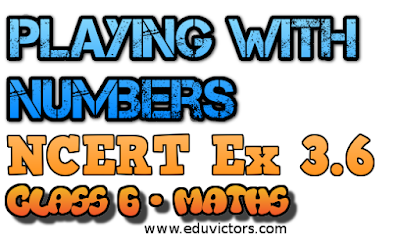## Saturday 29 April 2017

### CBSE Class 6 Maths - Playing With Numbers - NCERT Exercise 3.5 (#cbsenotes)

Playing With Numbers

## EXERCISE 3.5

CBSE Class 6 Maths

Q1: Which of the following statements are true?
(a) If a number is divisible by 3, it must be divisible by 9.
(b) If a number is divisible by 9, it must be divisible by 3.
(c) A number is divisible by 18, if it is divisible by both 3 and 6.
(d) If a number is divisible by 9 and 10 both, then it must be divisible by 90.
(e) If two numbers are co-primes, at least one of them must be prime.
(f) All numbers which are divisible by 4 must also be divisible by 8.
(g) All numbers which are divisible by 8 must also be divisible by 4.
(h) If a number exactly divides two numbers separately, it must exactly divide their sum.
(i) If a number exactly divides the sum of two numbers, it must exactly divide the two numbers separately.

(a) (e.g. 15 is divisible by 3 but not by 9)
(b)
(c)
(d)
(e) (e.g. 8 and 9 are co-primes and none of these are prime. )
(f)
(g)
(h)
(i)

Q2: Here are two different factor trees for 60. Write the missing numbers.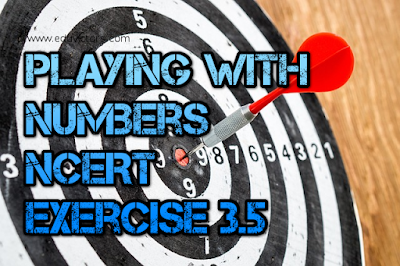## Playing With Numbers

EXERCISE 3.4
CBSE Class 6 Maths

Q1: Find the common factors of :
(a) 20 and 28
(b) 15 and 25
(c) 35 and 50
(d) 56 and 120

(a) Factors of 20 = 1, 2, 4, 5, 10, 20
Factors of 28 = 1, 2, 4, 7, 14, 28
Common factors = 1, 2, 4

(b) Factors of 15 = 1, 3, 5, 15
Factors of 25 = 1, 5, 25
Common factors = 1, 5

(c) Factors of 35 = 1, 5, 7, 35
Factors of 50 = 1, 2, 5, 10, 25, 50
Common factors = 1, 5

(d) Factors of 56 = 1, 2, 4, 7, 8, 14, 28, 56
Factors of 120 = 1, 2, 3, 4, 5, 6, 8, 10, 12, 15, 20, 24, 30, 60, 120
Common factors = 1, 2, 4, 8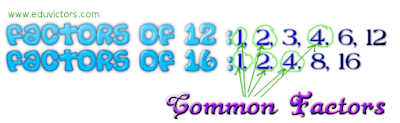## Playing With Numbers

EXERCISE 3.3
CBSE Class 6 Maths

Q1: Using divisibility tests, determine which of the following numbers are divisible by 2;
by 3; by 4; by 5; by 6; by 8; by 9; by 10 ; by 11 (say, yes or no):
```┌───────┬────────────────────────────────────────┐
│ Number│          Divisible By                  │
├───────┼───┬───┬────┬────┬────┬────┬────┬───┬───┤
│       │ 2 │ 3 │ 4  │ 5  │ 6  │ 8  │ 9  │ 10│11 │
├───────┼───┼───┼────┼────┼────┼────┼────┼───┼───┤
│ 128   │ Y │ N │ Y  │ N  │ N  │ Y  │ N  │ N │ ? │
├───────┼───┼───┼────┼────┼────┼────┼────┼───┼───┤
│ 990   │ ? │ ? │ ?  │ ?  │ ?  │ ?  │ ?  │ ? │ ? │
├───────┼───┼───┼────┼────┼────┼────┼────┼───┼───┤
│ 1586  │ ? │ ? │ ?  │ ?  │ ?  │ ?  │ ?  │ ? │ ? │
├───────┼───┼───┼────┼────┼────┼────┼────┼───┼───┤
│ 275   │ ? │ ? │ ?  │ ?  │ ?  │ ?  │ ?  │ ? │ ? │
├───────┼───┼───┼────┼────┼────┼────┼────┼───┼───┤
│ 6686  │ ? │ ? │ ?  │ ?  │ ?  │ ?  │ ?  │ ? │ ? │
├───────┼───┼───┼────┼────┼────┼────┼────┼───┼───┤
│639210 │ ? │ ? │ ?  │ ?  │ ?  │ ?  │ ?  │ ? │ ? │
├───────┼───┼───┼────┼────┼────┼────┼────┼───┼───┤
│429714 │ ? │ ? │ ?  │ ?  │ ?  │ ?  │ ?  │ ? │ ? │
├───────┼───┼───┼────┼────┼────┼────┼────┼───┼───┤
│ 2856  │ ? │ ? │ ?  │ ?  │ ?  │ ?  │ ?  │ ? │ ? │
├───────┼───┼───┼────┼────┼────┼────┼────┼───┼───┤
│ 3060  │ ? │ ? │ ?  │ ?  │ ?  │ ?  │ ?  │ ? │ ? │
├───────┼───┼───┼────┼────┼────┼────┼────┼───┼───┤
│406839 │ ? │ ? │ ?  │ ?  │ ?  │ ?  │ ?  │ ? │ ? │
└───────┴───┴───┴────┴────┴────┴────┴────┴───┴───┘

```## Playing With Numbers

EXERCISE 3.2
CBSE Class 6 MathsUlam Spiral (Arrange Numbers in Spiral and make Prime Number) credits: wikipedia user:onmywaybackhomeUlam Spiral (You see prime numbers appear at diagonals of rectangles) credit: wikipedia

Q1. What is the sum of any two
(a) Odd numbers?
(b) Even numbers?

(a) The sum of any two odd numbers is an even number.
Example:
1 + 3 = 4,
7 + 5 = 12
(b) The sum of any two even numbers is also an even number.
Example: 2 + 4 = 6,
8 + 18 = 26## Playing With Numbers NCERT Exercise 3.1

CBSE Class 6 Maths

Q1. Write all the factors of the following numbers :
(a) 24
(b) 15
(c) 21
(d) 27
(e) 12
(f) 20
(g) 18
(h) 23
(i) 36

(a) 24 = 1 × 24
= 2 × 12
= 3 × 8
= 4 × 6
= 6 × 4
∴ Factors of 24 = 1, 2, 3, 4, 6, 12, 24

(b) 15 = 1 × 15
= 3 × 5
= 5 × 3
∴ Factors of 15 = 1, 3, 5, 15

(c) 21 = 1 × 21
= 3 × 7
= 7 × 3
∴ Factors of 21 = 1, 3, 7, 21

(d) 27 = 1 × 27
= 3 × 9
= 9 × 3
∴ Factors of 27 = 1, 3, 9, 27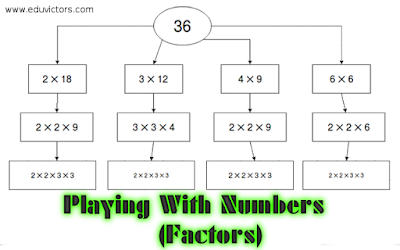##NCERT Exercise 2.3

CBSE Class 6 Maths - Chapter 2

Q1: Which of the following will not represent zero:

(a) 1 + 0
(b) 0 ✕ 0
(c)

 0 2

(d)
 10 - 10 2
(a) [∵ 1 + 0 = 1]

Q2: If the product of two whole numbers is zero, can we say that one or both of them will be zero? Justify through examples.## Whole Numbers - NCERT Ex 2.2

CBSE Class 6

Q1. Find the sum by suitable rearrangement:
(a) 837 + 208 + 363
(b) 1962 + 453 + 1538 + 647

(a) 837 + 208 + 363
= (837 + 363) + 208
= 1200 + 208
= 1408

(b) 1962 + 453 + 1538 + 647
= (1962 + 1538) + (453 + 647)
= 3500 + 1100
= 4600

Q2. Find the product by suitable rearrangement:
(a) 2 × 1768 × 50
(b) 4 × 166 × 25
(c) 8 × 291 × 125
(d) 625 × 279 × 16
(e) 285 × 5 × 60
(f) 125 × 40 × 8 × 25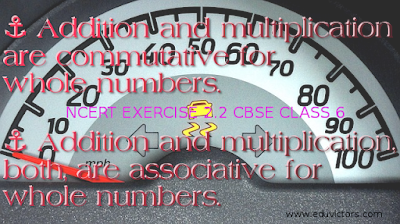## Chapter 2: Whole Numbers

NCERT Ex 2.1

CBSE Class 6 Maths

Q1: Write the next three natural numbers after 10999.

10,999 + 1 = 11,000
11,000 + 1 = 11,001
11,001 + 1 = 11,002

Q2: Write the three whole numbers occurring just before 10001.

10,001 – 1 = 10,000
10,000 – 1 = 9,999
9,999  – 1 = 9,998

Q3: Which is the smallest whole number?

Answer: ‘0’ (zero) is the smallest whole number.## Saturday 1 April 2017

### CBSE Class 6 - Maths - CH1 - Knowing Your Numbers (NCERT Ex 1.3) (#cbsenotes)

CBSE Class 6 - Maths - CH1 - NCERT Ex 1.3 Answers

Q1. Estimate each of the following using general rule:
(a) 730 + 998
(b) 796 – 314
(c) 12,904 +2,888
(d) 28,292 – 21,496

(a) 730 round off to     700
998 round off to 1000
──────
Estimated sum =  1700
──────

(b) 796 round off to   800
314 round off to 300
──────
Estimated sum = 500
──────

(c) 12904 round off to 13000
2888 round off to   3000
──────
Estimated sum  = 16000
──────

(d) 28292 round off to      28000
21496 round off to  21000
──────
Estimated difference = 7000
──────## Knowing Our Numbers

NCERT Ex 1.2 Solutions

CBSE Class 6 Maths

Q1. A book exhibition was held for four days in a school. The number of tickets sold at the counter on the first, second, third and final day was respectively 1094, 1812, 2050 and 2751. Find the total number of tickets sold on all the four days.

Number of tickets sold on first day = 1,094
Number of tickets sold on second day = 1,812
Number of tickets sold on third day = 2,050
Number of tickets sold on fourth day = 2,751
────────
Total tickets sold = 7,707
────────
∴ 7,707 tickets were sold on all the four days.

Q2: Shekhar is a famous cricket player. He has so far scored 6980 runs in test matches. He wishes to complete 10,000 runs. How many more runs does he need?